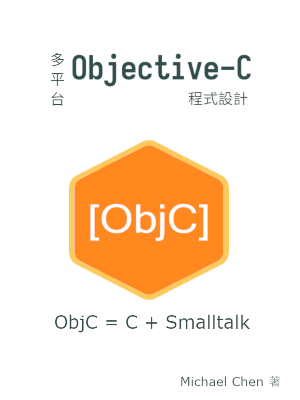# 用 Objective-C++ 混合 C++ 和 Objective-C 程式碼

## 前言

除了直接用 Objective-C 實作程式以外，Objective-C 還可以用來橋接 C 函式庫。因為 Objective-C 基本上是 C 的嚴格超集，可以直接引用外部 C 函式庫，再包上一層 Objective-C 類別。

除了 C 以外，C++ 是另一個有龐大生態圈的 C 家族語言。如果我們想要使用外部 C++ 函式庫，我們不需要為該函式庫寫 C 的 binding，因為藉由 Objective-C++ 我們可以直接橋接 C++ 函式庫。

Objective-C++ 並不是新的語言，而是容許 Objective-C 和 C++ 程式碼混合撰寫的模式。由於 Objective-C 的部分和原本的語法相同，我們只要加上和 C++ 相關的部分即可。本文會以兩個簡短範例來說明如何撰寫 Objective-C++ 程式。

## 撰寫第一隻 Objective-C++ 程式

依照慣例，我們用 Objective-C++ 寫經典的 Hello World 程式。可參考以下程式碼：

``````/* hello.mm */
#import <Foundation/Foundation.h>
#include <iostream>

using std::cout;
using std::endl;

int main(void)
{
NSAutoreleasePool *pool = \
[[NSAutoreleasePool alloc] init];
if (!pool)
return 1;

cout << "Hello C++" << endl;

NSLog(@"Hello Objective-C\n");

[pool drain];

return 0;
}
``````

這隻程式的行為相當簡單，只是分別用 C++ 和 Objective-C 的標準輸出印出字串而已。本程式可正確編譯、執行，代表我們可以把 Objective-C 和 C++ 程式碼混合在一起。

請注意我們在這裡使用 Objective-C 的記憶體池來管理記憶體，因為我們考量 Clang 和 GCC 間的編譯器相容性。至於 C++ 的部分沒有用到手動記憶體配置，所以不需手動釋放記憶體。

## 編譯第一隻 Objective-C++ 程式

承上節，我們來看如何編譯 Objective-C++ 程式。

按照慣例，Objective-C++ 的程式碼的副檔名會以 .mm 而非 .m 結尾，用來提示編譯器要以 Objective-C++ 模式來編譯程式碼。以本例來說，我們將檔案命名為 hello.mm

此外，我們應該用 C++ 編譯器而非 C 編譯器來編譯 Objective-C++ 原始碼，因為 C 編譯器無法解析 C++ 多出來的語法。

雖然 Objecitve-C 沒有 ISO 標準，目前實質的標準應當是 Mac 平台的 Clang 編譯器。我們以 Clang 的 C++ 編譯器 Clang++ 來編譯此範例程式：

``````\$ clang++ -o hello hello.mm -lobjc -framework Foundation
``````

如果在非 Mac 平台上，可還擇 GCC 或 Clang 所附的 C++ 編譯器。以下指令用 `g++` 編譯此範例程式：

``````\$ g++ -o hello hello.mm -lobjc -lgnustep-base -I /usr/include/GNUstep -L /usr/lib/GNUstep -fconstant-string-class=NSConstantString
``````

說實在的，指令有點長。因為 GNUstep 通常不是位於類 Unix 系統的標準位置，所以我們要自行加上路徑相關的編譯參數。

如果使用 `clang++` 來編譯，指令又更長一些：

``````\$ clang++ -o hello hello.mm -lobjc -lgnustep-base -I /usr/include/GNUstep -L /usr/lib/GNUstep -I /usr/lib/gcc/x86_64-linux-gnu/7.4.0/include -fconstant-string-class=NSConstantString
``````

這是因為 objc/objc.h 的位置不在 Clang 的預設位置，所以要手動加入。指令內的 GCC 版本號會因系統而異，請讀者不要死背指令。其實我們在這裡借用 GCC 所附的標頭檔，基本上可以正常編譯。

## 用 Objective-C++ 包裝 C++ 類別

Objective-C++ 的主要意義不在於 Objective-C 和 C++ 的混合編碼，而是橋接 C++ 類別。因為我們在專案中混入 Objective-C++ 程式碼後，整個專案被迫要以 C++ 來寫。一般來說，我們會希望主程式仍保持純 Objective-C 的模式，只在類別中混入 Objective-C++ 的程式碼。

如果團隊成員協調好，大家都願意用 Objective-C++ 模式來寫的話，在內部用程式碼中混用 C++ 程式就無所謂。反之，如果是要對外發佈的公開函式庫，則應保持純 Objective-C 的模式。

在本節範例中，我們假定先前已經寫好了代表平面座標的 `Point` 類別，現在要為 `Point` 類別加上 Objective-C 的 binding，所以我們會用到 Objective-C++ 模式來寫程式碼。

我們先來看 `Point` 類別的公開宣告：

``````/* point.hpp */
#pragma once

class Point
{
public:
Point(double x, double y);
double x();
double y();
static double distance(Point *p, Point *q);
private:
double _x;
double _y;
};
``````

`Point` 類別的宣告相當簡單，不多做說明。

再來看 `Point` 類別內部的實作：

``````/* point.cpp */
#include <cmath>
#include "point.hpp"

Point::Point(double x, double y)
{
this->_x = x;
this->_y = y;
}

double Point::x()
{
return this->_x;
}

double Point::y()
{
return this->_y;
}

double Point::distance(Point *p, Point *q)
{
double dx = p->x() - q->x();
double dy = p->y() - q->y();

return sqrt(pow(dx, 2) + pow(dy, 2));
}
``````

同樣地，`Point` 類別內部的實作也相當簡單，不另做說明。

我們來看 `ObjPoint` 的公開界面宣告：

``````/* objpoint.h */
#pragma once

#import <Foundation/Foundation.h>

#ifdef __cplusplus
extern "C" {
#endif

@interface ObjPoint : NSObject {
void *p;
}

-(ObjPoint *) init;
-(ObjPoint *) initWithX: (double) x andY: (double) y;

-(void) dealloc;

+(double) distanceBetween: (ObjPoint *) p andPoint: (ObjPoint *) q;

-(double) x;
-(double) y;
@end

#ifdef __cplusplus
}
#endif
``````

請注意此宣告是純 Objective-C 而非 Objective-C++。取巧的地方是用 `void *` 這個 opaque pointer 來宣告內部物件的型別，所以不會用到 C++ 的語法。

我們接著來看 `ObjPoint` 內部的實作：

``````/* objpoint.mm */
#include <cmath>
#include "point.hpp"
#import "objpoint.h"

@implementation ObjPoint
-(ObjPoint *) init
{
return [self initWithX: 0.0 andY: 0.0];
}

-(ObjPoint *) initWithX: (double)x andY: (double)y
{
if (!self)
return self;

self = [super init];

p = (void *) new Point(x, y);
if (!p) {
[super release];
self = nil;
return self;
}

return self;
}

-(void) dealloc
{
if (!self)
return;

delete (Point *) p;
[super dealloc];
}

+(double) distanceBetween: (ObjPoint *)p andPoint: (ObjPoint *)q
{
return Point::distance((Point *) (p->p), (Point *) (q->p));
}

-(double) x
{
return ((Point *) p)->x();
}

-(double) y
{
return ((Point *) p)->y();
}
@end
``````

`ObjPoint` 的內部實作中，真正的運算並非由 `ObjPoint` 類別來負責，而是由 `Point` 類別來負責。`ObjPoint` 類別只是用來包住型別為 `Point *` 的物件 `p`，並提供適用於 Objective-C 的公開界面。

由於我們把 `p` 宣告成 `void *` 型別，在訊息內部我們得手動轉型，才能使用 `Point` 類別所提供的方法。

此外，我們有用到 `new` 來配置物件 `p` 的記憶體，所以要在 `release` 訊息中以 `delete` 手動釋放記憶體。

假定我們的外部程式也用 Objective-C++ 來寫。可參考以下範例程式：

``````/* main.mm */
#import <Foundation/Foundation.h>
#include <iostream>
#import "objpoint.h"

using std::cerr;
using std::endl;

int main(void)
{
NSAutoreleasePool *pool = [[NSAutoreleasePool alloc] init];
if (!pool)
return 1;

ObjPoint *p;
ObjPoint *q;

p = [[[ObjPoint alloc] init] autorelease];
if (!p) {
cerr << "Failed to allocate p" << endl;
goto ERROR;
}

q = [[[ObjPoint alloc] initWithX: 3.0 andY: 4.0] autorelease];
if (!q) {
cerr << "Failed to allocate q" << endl;
goto ERROR;
}

if (!(5.0 == [ObjPoint distanceBetween: p andPoint: q])) {
cerr << "Wrong distance" << endl;
goto ERROR;
}

[pool drain];

return 0;

ERROR:
[pool drain];

return 1;
}
``````

這個範例沒有什麼困難的地方，最大的特點是 Objective-C 和 C++ 程式碼在同一個檔案中混在一起。

假定我們的外部程式想用純 Objective-C。可將上例改寫如下：

``````/* main.m */
#import <Foundation/Foundation.h>
#include <stdio.h>
#import "objpoint.h"

int main(void)
{
NSAutoreleasePool *pool = [[NSAutoreleasePool alloc] init];
if (!pool)
return 1;

ObjPoint *p;
ObjPoint *q;

p = [[[ObjPoint alloc] init] autorelease];
if (!p) {
fprintf(stderr, "Failed to allocate p\n");
goto ERROR;
}

q = [[[ObjPoint alloc] initWithX: 3.0 andY: 4.0] autorelease];
if (!q) {
fprintf(stderr, "Failed to allocate q\n");
goto ERROR;
}

if (!(5.0 == [ObjPoint distanceBetween: p andPoint: q])) {
fprintf(stderr, "Wrong distance\n");
goto ERROR;
}

[pool drain];

return 0;

ERROR:
[pool drain];

return 1;
}
``````

其實兩個範例在本質上是相同的，差別只在要用 Objective-C 還是 Objective-C++ 模式來寫。理想上的 Objective-C 類別，不應強迫外部程式使用 Objective-C++ 模式來寫，所以我們在這個範例程式中刻意使用兩個不同的外部程式來執行此類別。

## 使用 GCC 編譯 `Point` 程式

在上一節的範例中，我們混合了 C++、Objective-C、Objective-C++ 三種語言的原始碼，所以不適合用單一指令來編譯。在本節中，我們以 GCC 為例，將編過程拆解，讓讀者了解如何編譯混合多種語言的原始碼。

我們會先將原始碼編譯目的檔，再由目的檔編譯成可執行檔。藉由這種方式，我們可以把編譯的過程拆開，對不同類型的檔案用不同的指令來編譯。

point.cpp 是 C++ 原始碼，使用一般的指令來編譯即可：

``````\$ g++ -c -o point.o point.cpp
``````

objpoint.mm 是 Objective-C++ 原始碼，所以要加上必要的參數：

``````\$ g++ -c -o objpoint.o objpoint.mm -I /usr/include/GNUstep -fconstant-string-class=NSConstantString
``````

假定我們的主程式也用 Objective-C++ 來寫，同樣要加上相關參數：

``````\$ g++ -c -o main.o main.mm -I /usr/include/GNUstep -fconstant-string-class=NSConstantString
``````

最後再將所有的目的檔一起編成執行檔即可。同樣要加上相關參數：

``````\$ g++ -o point point.o objpoint.o main.o -lobjc -lgnustep-base -I /usr/include/GNUstep -L /usr/lib/GNUstep -fconstant-string-class=NSConstantString
``````

如果我們的主程式用 Objective-C 來寫，則改用 C 編譯器來編譯：

``````\$ gcc -c -o main.o main.m -I /usr/include/GNUstep -fconstant-string-class=NSConstantString
``````

這時候，我們可以繼續用 C 編譯器來編譯。但程式中有用到 C++ 的部分，所以要額外加上 `-lstdc++` 來連結 C++ 標準函式庫：

``````\$ gcc -o point point.o objpoint.o main.o -lstdc++ -lm -lobjc -lgnustep-base -I /usr/include/GNUstep -L /usr/lib/GNUstep -fconstant-string-class=NSConstantString
``````

透過這樣的流程，就可以編譯此範例程式。

## 使用 Clang 編譯 `Point` 程式

除了 GCC 外，Clang 也是 Objective-C 編譯器。基本上 Clang 和 GCC 的參數是相容的，但在非 Mac 平台編譯 Objective-C 程式時，需要額外加入標頭檔 objc/objc.h 所在的位置。以下是實例：

``````\$ clang++ -o point point.o objpoint.o main.o -lobjc -lgnustep-base -I /usr/include/GNUstep -L /usr/lib/GNUstep -I /usr/lib/gcc/x86_64-linux-gnu/7.4.0/include -fconstant-string-class=NSConstantString
``````

由於 Clang 和 GCC 大部分參數相容，故我們不重覆列出指令。

## 附註：快速地編譯專案程式碼

雖然用指令手動編譯 Objective-C 程式是很好的學習，重覆輸入久了也會感到厭煩。正規的方法是寫 Makefile 或其他的編譯設定檔。但每次練習都要寫 Makefile，其實沒有省到什麼時間。

著眼於這項議題，筆者撰寫了 objcheck，這是一個小型 shell 命令稿，可在不使用外部編譯設定檔的前提下，快速編譯 Objective-C 程式。本程式相容於 C、C++、Objective-C、Objective-C++ 等會在 Objective-C 專案中出現的程式語言。有興趣的讀者可前往 `objcheck` 的專案頁面，也可以自由地取用該命令稿。

## 評語

比起 Objective-C 來說，Objective-C++ 的能見度就低得多。蘋果公司甚至移除了官網上和 Objective-C++ 相關的頁面，只能在網路上找到一些零散的文章。或許這表達了蘋果公司不希望大家使用 Objective-C++ 的態度。

Objective-C++ 其實有很多本文未提及的限制，像是 Objective-C 的類別和 C++ 的類別不能互相繼承，Objective-C 的類別不能用命名空間等。C++ 沒有辦法解放 Objective-C，反而是 Objective-C 限制了某些 C++ 的特性。

縇合上述現象，我們應該把 Objective-C++ 視為橋接 C++ 程式碼的工具，並減少 Objective-C++ 對整個 Objective-C 專案的影響。而不應該直接在 Objective-C 專案中大量混合 Objective-C 和 C++ 程式碼。

## 電子書籍

如果你覺得這篇 Objective-C 的技術文章對你有幫助，可以看看這本 Objective-C 程式設計電子書：【分享本文】